NCERT Exemplar - MCQs

Chapter 5 Class 12 Continuity and Differentiability
Serial order wise

## (D) 3π/4

This question is similar to Ex 5.8, 1 - Chapter 5 Class 12 - Continuity and Differentiability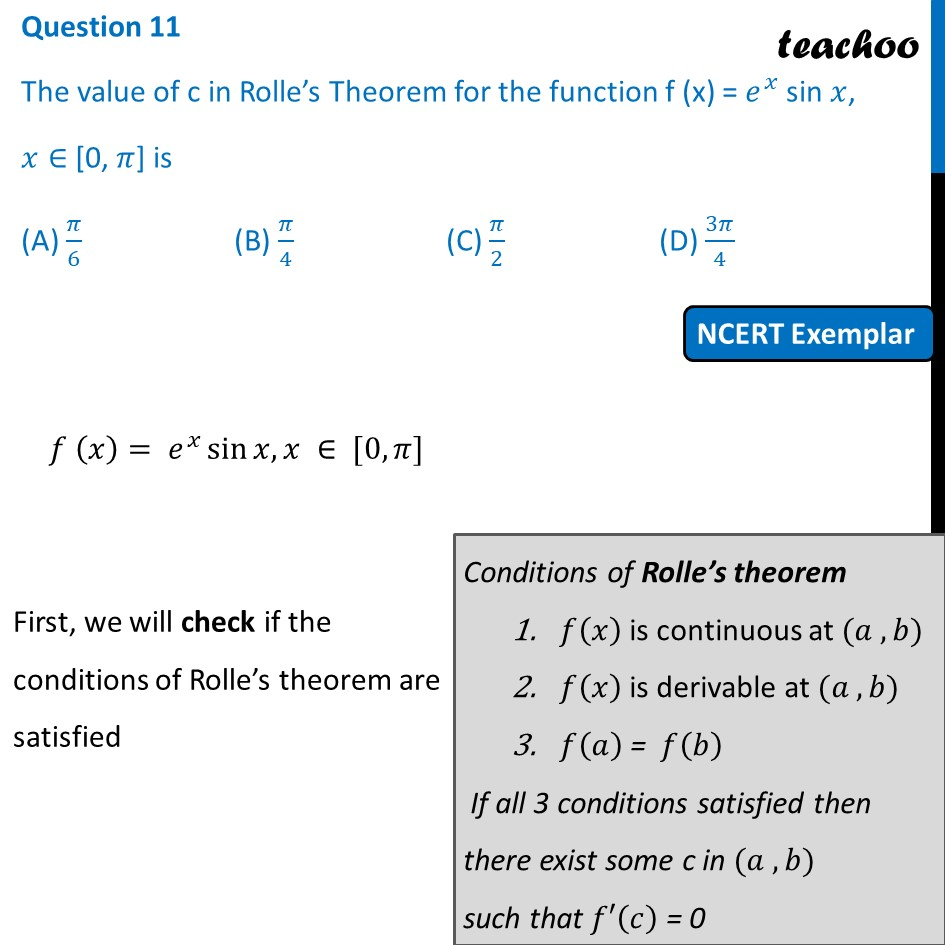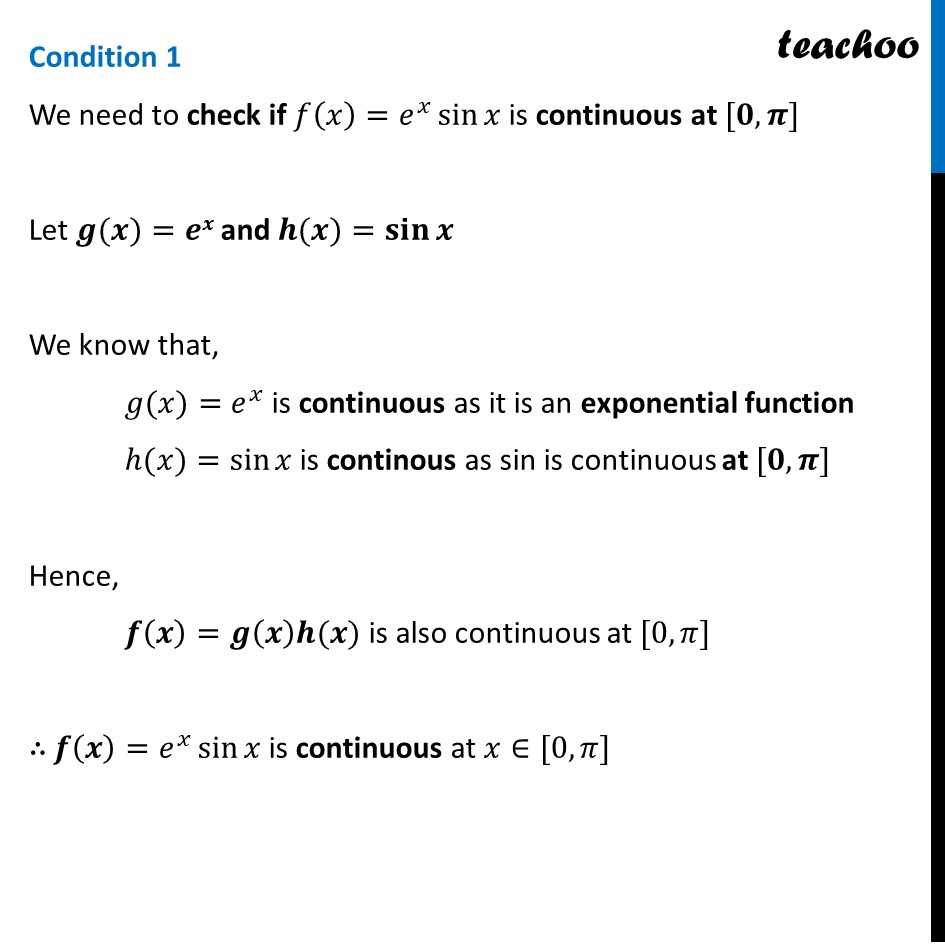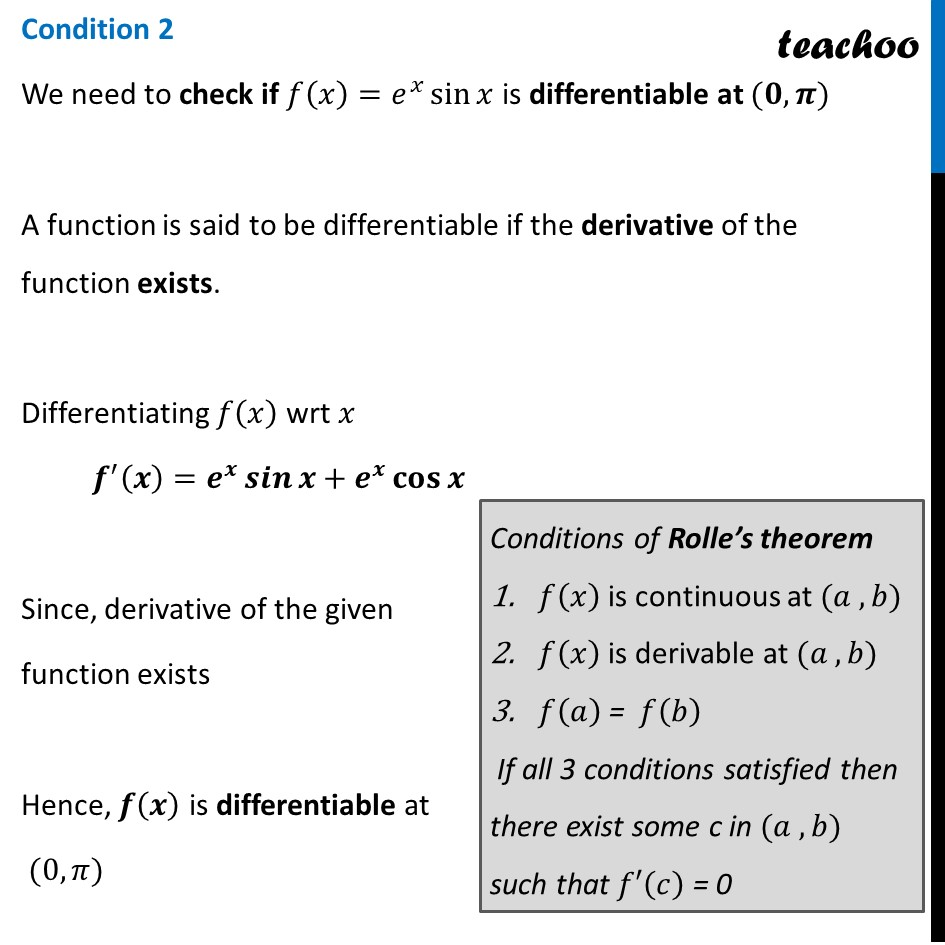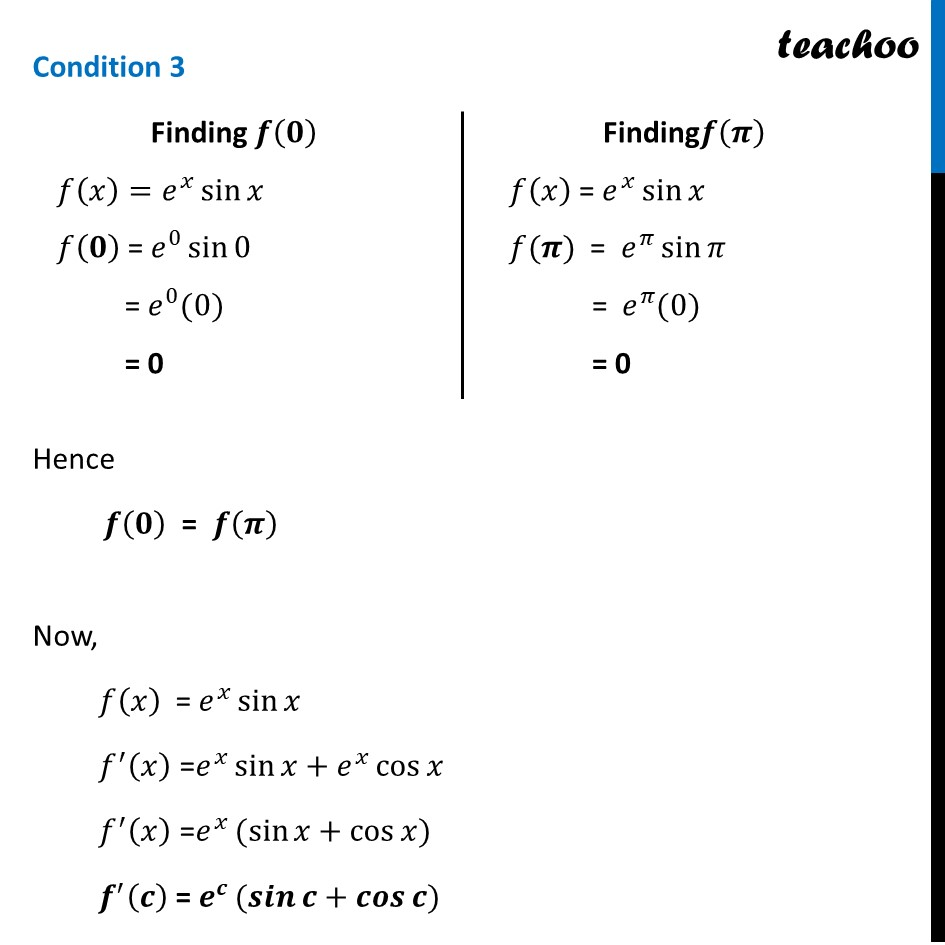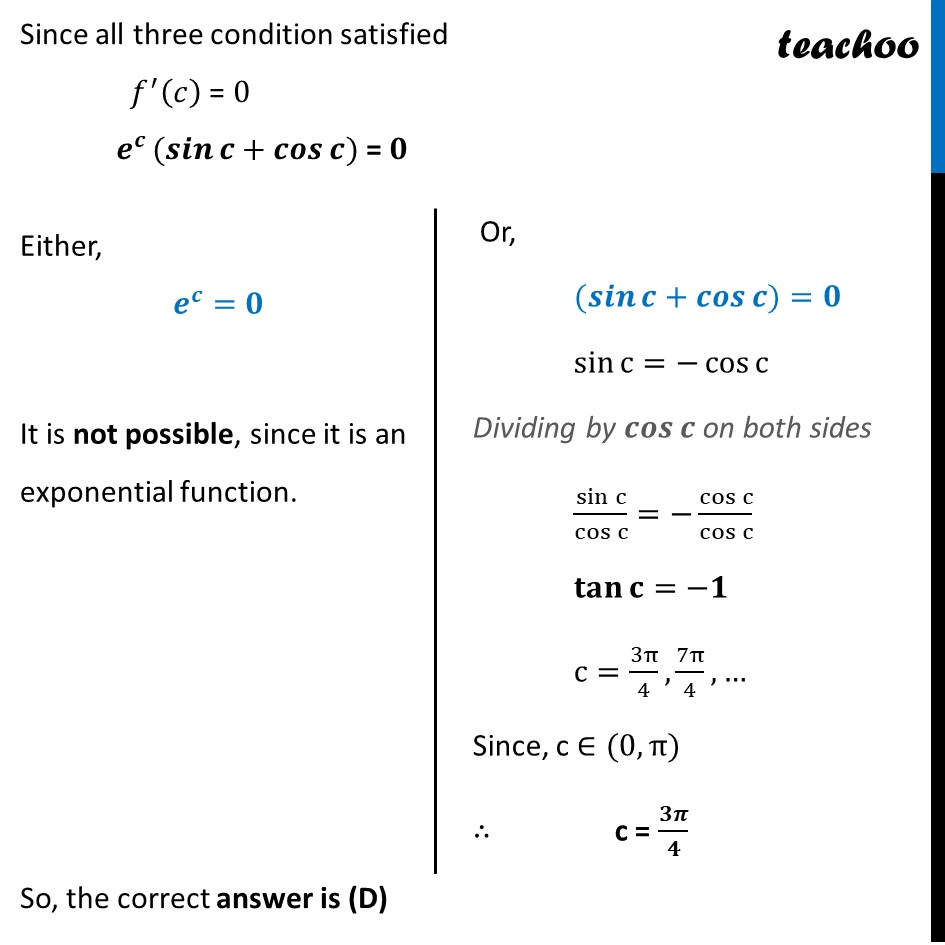Learn in your speed, with individual attention - Teachoo Maths 1-on-1 Class

### Transcript

Question 1 The value of c in Rolle’s Theorem for the function f (x) = 𝑒^𝑥 sin 𝑥, 𝑥∈ [0, 𝜋] is (A) 𝜋/6 (B) 𝜋/4 (C) 𝜋/2 (D) 3𝜋/4 𝑓 (𝑥)= 𝑒^𝑥 sin⁡〖𝑥, 𝑥 ∈ [0,𝜋] 〗 First, we will check if the conditions of Rolle’s theorem are satisfied Condition 1 We need to check if 𝑓(𝑥)=𝑒^𝑥 sin⁡〖𝑥 〗is continuous at [𝟎,𝝅] Let 𝒈(𝒙)=𝒆𝒙 and 𝒉(𝒙)=𝐬𝐢𝐧⁡𝒙 We know that, 𝑔(𝑥)=𝑒^𝑥 is continuous as it is an exponential function ℎ(𝑥)=sin⁡𝑥 is continous as sin is continuous at [𝟎,𝝅] Hence, 𝒇(𝒙)=𝒈(𝒙)𝒉(𝒙) is also continuous at [0,𝜋] ∴ 𝒇(𝒙)=𝑒^𝑥 sin⁡𝑥 is continuous at 𝑥∈[0,𝜋] Condition 2 We need to check if 𝑓(𝑥)=𝑒^𝑥 sin⁡𝑥 is differentiable at (𝟎,𝝅) A function is said to be differentiable if the derivative of the function exists. Differentiating 𝑓(𝑥) wrt 𝑥 𝒇^′ (𝒙)=𝒆^𝒙 𝒔𝒊𝒏⁡𝒙+𝒆^𝒙 𝐜𝐨𝐬⁡𝒙 Since, derivative of the given function exists Hence, 𝒇(𝒙) is differentiable at (0,𝜋) Finding 𝒇(𝟎) 𝑓(𝑥)=𝑒^𝑥 sin⁡𝑥 𝑓(𝟎) = 𝑒^0 sin⁡0 = 𝑒^0 (0) = 0 Finding𝒇(𝝅) 𝑓(𝑥) = 𝑒^𝑥 sin⁡𝑥 𝑓(𝝅) = 𝑒^𝜋 sin⁡𝜋 = 𝑒^𝜋 (0) = 0 Condition 3 Hence 𝒇(𝟎) = 𝒇(𝝅) Now, 𝑓(𝑥) = 𝑒^𝑥 sin⁡𝑥 𝑓^′ (𝑥) =𝑒^𝑥 sin⁡𝑥+𝑒^𝑥 cos⁡𝑥 𝑓^′ (𝑥) =𝑒^𝑥 〖(sin〗⁡𝑥+cos⁡𝑥) 𝒇^′ (𝒄) = 𝒆^𝒄 〖(𝒔𝒊𝒏〗⁡𝒄+𝒄𝒐𝒔⁡𝒄) Since all three condition satisfied 𝑓^′ (𝑐) = 0 𝒆^𝒄 〖(𝒔𝒊𝒏〗⁡𝒄+𝒄𝒐𝒔⁡𝒄) = 𝟎 Either, 𝒆^𝒄=𝟎 It is not possible, since it is an exponential function. Or, 〖(𝒔𝒊𝒏〗⁡𝒄+𝒄𝒐𝒔⁡𝒄)=𝟎 sin⁡c=−cos⁡c Dividing by 𝒄𝒐𝒔⁡𝒄 on both sides sin⁡c/cos⁡c =−cos⁡c/cos⁡c 𝐭𝐚𝐧⁡〖𝐜=−𝟏〗 c=3π/4, 7π/4, … Since, c ∈(0,π) ∴ c = 𝟑𝝅/𝟒 So, the correct answer is (D)